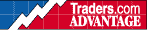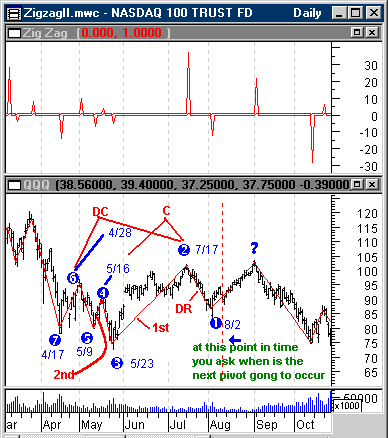HOT TOPICS LIST

INDICATORS LIST

LIST OF TOPICS

# Swing Prediction (Part II of III)

11/19/01 04:25:08 PM
by Dennis D. Peterson

Here is a system that incorporates swing strength with time.

Security:   QQQ
Position:   N/A

 Part I... Part II... Part III Robert Miner, in his article "A Spreadsheet For Time Ratio Analysis" (Dec. 1992, 'Technical Analsysis of Stocks & Commodities') devised a system that incorporated swing strength with time. I have tried to encode it with Metastock, and I don't believe it can be entirely encoded but I'll show what I was able to do. If I had been able to fully encode his algorithm I would want to play with cycle definition and use some other ratios. There is, however, an Excel spreadsheet which I put in Part III.Figure 3: Bars Per Swing (top chart) and Daily QQQ (bottom chart). Top chart is an indicator that shows on the positive side how many bars have just been spent in an upswing and on the negative side how many bars have been spent on the downside. The bottom chart is daily QQQ price for 2000. The circled numbers are the pivots that are used to define the swings that will be used for predicting when ? will occur. Graphic provided by: MetaStock. Graphic provided by: Data vendor: eSignal<. I looked at QQQ price history in 2000 and wanted to see if I could get a prediction of when the next pivot or swing point would occur after 8/2. Would I get a prediction of around 8/31 or 9/1? There are a number of features that are attractive about Miner's approach. First, he uses swing duration and time. Second, to project forward in time, he uses Fibonacci ratios. I have used Fibonacci time cycle projections (1, 2, 3, 5, 8, 13, 21, . . .days in the future) in the past, and while I have been underwhelmed with their performance, if I had a choice it would be the first place I would look. The very modest amount of success I have seen occurs when I find an equity that has gone through a major peak or valley and putting the start of the Fibonacci time cycle start on the peak or valley to predict future swing points. Third, I have always thought that hitting any date is a matter of chance and what I want is something that would somehow say this date has better odds than another date, and Miner does this by looking for date clusters. Figure 3 (bottom chart) has been annotated with a series of circled numbers to identify swing points. What Miner wants to do is use the dates of the last two swing points, in this case 8/2 and 7/17, to add on days for a prediction. I have excerpted Miner's rules below and have only changed the figure reference to fit. I have annotated the chart with what Miner calls DR, first (1st) and second (2nd) alternates waves, prior cycle (C), and prior dual cycle (DC). The definition follows:1. Direct time retracements (DR): The most recent cycle or the swing into the last pivot point. I call the forward dates from this cycle direct time retracements. In Figure 3, this would be the number of days from point 2 to point 1 and then projected forward from point 1.2. The prior two alternate cycles (1st and 2nd alt): These are the prior two swings in the same direction of the current market direction from the last pivot point. The last two alternate cycles are proportioned and the projections made from the last swing point. In Figure 3, the first alternate cycle is the day count from point 3 to point 2 and projected forward from point 1. The second alternate cycle is the daycount from point 5 to point 4 and projected from point 1.3. The prior cycle (C): If the last pivot point was a low, the prior cycle would be the last high - high. Ifthe last pivot point was a high, the prior cycle would be the last low - low . In Figure 3, this would bethe day counts between points 4 and 2 and projected from 2.4. The prior dual cycle (DC): If the last pivot point was a low, the prior dual cycle would be the periodfrom the last high to the third high back or the dual or double cycle of similar degree. From a highpivot, the prior dual cycle would be the period from the last to the third prior low. In Figure 3, thiswould be the day count from point 6 to point 2 and projected from point 2.What Miner will do is use two ratios for each projection, and always project from either 7/17 or 8/2. Because the spreadsheet used calendar days and I wanted to use trading days only, I made some calculations by hand and put them in the notepad capture below. The format below shows the ratios used and each left adjusted line is read as cycle name, (swing points used), date of swing, how many days in swing, (the two ratios to use). For example the first line says this is direct retracement (DR), going from swing 1 to swing 2, with swing 1 ending on 8/02, which was a swing down of 12 trading days long, and then to multiply by the ratios of .382 and 1.618. Calculations used when looking for a high pivot: DR (1-2): 8/02 12 days down (use .382 and 1.618)12 x .382 = 4.584 days forward (8/10)12 x 1.618 = 19.416 days forward (8/29)1st alt (3-2): 7/17 37 days up (use .5 and 1.732)37 x .5 = 18.5 days forward (8/11)37 x 1.732 = 64.08 days forward (10/16)2nd alt (5-4): 5/16 4 days up (use .618 and 2)4 x .618 = 2.4 days forward (5/18)4 x 2 = 8 days forward (5/26)C (4-2): 7/17 42 day cycle (.707 and 2.618)42 x .707 = 29.69 days forward (8/28)42 x 2.618 = 109.96 days forward (approximately 11/21)DC (6-2): 7/17 53 day cycle (use 1 and 3)53 x 1 = 53 days forward (9/27)53 x 3 = 159 days forward (approximately 1/24/2001)You can see that 8/28 and 8/29 are two dates close together, as well as 8/10 and 8/11. It turns out that 8/10 and 8/11 were reaction days, but then hitting a reaction day is a bit akin to hitting a barn with a shotgun blast. In Part III you will see 50+ dates. So here are ten manual calculations I captured with notepad versus the 50+ using Excel, and a small cluster of two at 8/28 and 8/29 that appear with just 10. This gets us to a place where additional analysis may pay off. Part I... Part II... Part III

Dennis D. Peterson

Market index trading on a daily basis.

 Comments or Questions? Article Usefulness 5 (most useful) 4 3 2 1 (least useful)

Date:�11/27/01Rank:�4Comment: